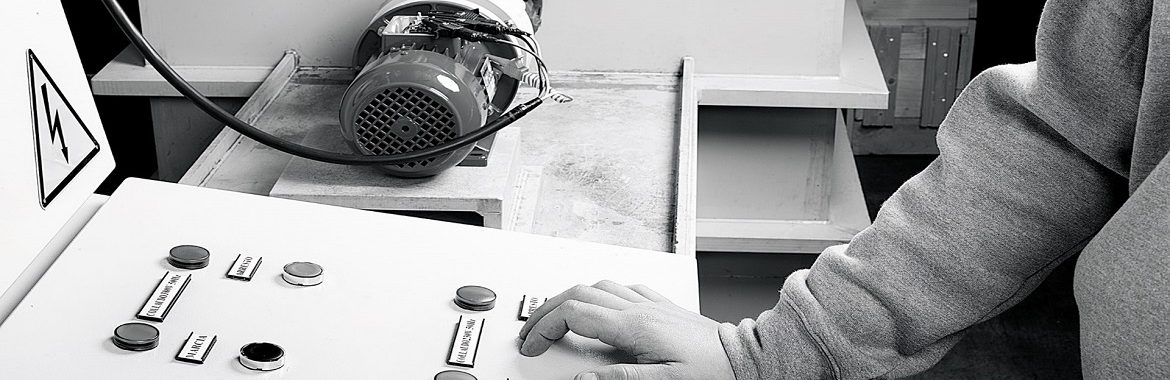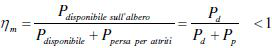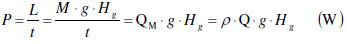# PUMP POWER RATINGTo define the pump power rating we must introduce certain necessary parameters.

## PUMP PERFORMANCE

### MECHANICAL PUMP PERFORMANCE

This value takes into account the friction between the mechanical parts of the pump.Usually it has values between 0.88 and 0.96, which are rather high. After all the reduction of friction is one of the key targets to safeguard the life and reliability of the pump.

### ELECTRIC PUMP PERFORMANCE

This value represents the efficiency of the electric motor, usually around 0.9.

### VOLUMETRIC PUMP PERFORMANCE

The volumetric performance of a pump is used to quantify the losses of fluid volume due to the play between pump impeller and casing. The values are usually between 0.9 and 0.96.

## CALCULATING PUMP POWER RATING

Once these values are defined the total efficiency of the pump will be given by the result of the various efficiencies:

ηt = ηm * ηel * ηvol

Now we can calculate the power of the pump.

Theoretically the power is defined as the work that the machine must perform to move a mass of fluid in the unit of time.

The formula for the power of a pump is:L = work (joule)
g = gravity acceleration (m/s2)
ρ = fluid density (kg/dm3)
t = time (s)
Hm = overall losses from the plant (m)
M = mass (kg)